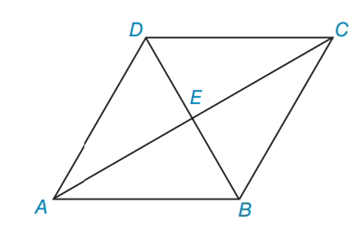Chapter 4.3, Problem 16E### Elementary Geometry for College St...

6th Edition
Daniel C. Alexander + 1 other
ISBN: 9781285195698

#### Solutions

Chapter
Section### Elementary Geometry for College St...

6th Edition
Daniel C. Alexander + 1 other
ISBN: 9781285195698
Textbook Problem
78 views

# In Exercises 15 to 18, consider rhombus ABCD with diagonals A C ¯  and  D B ¯ . When the answer is not a whole number, leave a square root answer.Exercises 15 – 18If AE = 6 and EB = 5, find AB.

To determine

To find:

AB of the given rhombus.

Explanation

The basic properties of rhombus:

1) All the properties of a parallelogram apply (the ones that matter here are parallel sides, opposite angles are congruent, and consecutive angles are supplementary).

2) All sides are congruent by definition.

3) The diagonals bisect the angles.

4) The diagonals are perpendicular bisectors of each other.

Calculation:

Given: AE = 6 and EB = 5

Consider the rhombus.

To find AB:

From the property, in rhombus, diagonals are perpendicular bisectors.

In rhombus ABCD, AEB is right triangle.

By using Pythagorean Theorem

### Still sussing out bartleby?

Check out a sample textbook solution.

See a sample solution

#### The Solution to Your Study Problems

Bartleby provides explanations to thousands of textbook problems written by our experts, many with advanced degrees!

Get Started

#### In Exercises 4562, find the values of x that satisfy the inequality (inequalities). 60. 2x3x14

Applied Calculus for the Managerial, Life, and Social Sciences: A Brief Approach

#### 30. If a variable x is normally distributed, with and

Mathematical Applications for the Management, Life, and Social Sciences

#### Simplify: 3064

Elementary Technical Mathematics

#### Differentiate. f(x) = (3x2 5x)ex

Single Variable Calculus: Early Transcendentals

#### The graph of x = cos t, y = sin2 t is:

Study Guide for Stewart's Single Variable Calculus: Early Transcendentals, 8th

#### Identify and describe the steps in the research process.

Research Methods for the Behavioral Sciences (MindTap Course List)